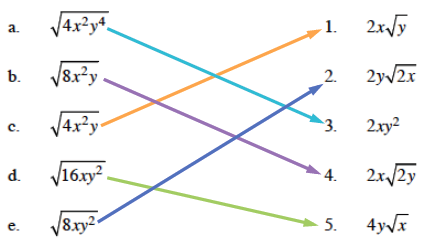### Home > CCA2 > Chapter Ch3 > Lesson 3.1.1 > Problem3-6

3-6.

Match each expression on the left with its equivalent expression on the right. Assume that all variables represent positive values. Be sure to justify how you know each pair is equivalent. Homework Help ✎

1. $\sqrt { 4 x ^ { 2 } y ^ { 4 }}$

1. $2x\sqrt{y}$

1. ${ \sqrt { 8 x ^ { 2 } y } }$

1. $2y\sqrt{2x}$

1. ${ \sqrt { 4 x ^ { 2 } y } }$

1. $2xy^2$

1. ${ \sqrt { 16 x y ^ { 2 } } }$

1. $2x\sqrt{2y}$

1. ${ \sqrt { 8 x y ^ { 2 } }}$

1. $4y\sqrt{x}$

Simplify the expressions in the left column. For example:

$\sqrt{8\textit{xy}^2} = \sqrt{4 \cdot 2 \cdot \textit{x} \cdot \textit{y}^2}=\sqrt{4} \cdot \sqrt{\textit{y}^2} \cdot \sqrt{2\textit{x}}$

Take the square roots of $4$ and $y^2$.